• 基于Matlab平台的瑞雷波频散曲线提取
2020-12-21 12:52:50

龙源期刊网

http://www.qikan.com.cn

基于

Matlab

平台的瑞雷波频散曲线提取

作者：吴少奇

刘雪峰

甘立烽

柳承成

来源：《科技视界》

2016

年第

26

【摘

要】拉东变换算法是目前从瑞雷波中提取频散曲线的较常用和较好的方法。由于拉

东变换算法复杂，给提取频散曲线带来不便；为了提高提取效率和优化提取效果，本文利用

Matlab

软件平台对该算法进行可视化的实现，为利用频散曲线进行相关研究提供便捷。

【关键词】瑞雷波；频散曲线；拉东变换；

Matlab

Abstract

The arithmetic of Radon Transform is a common and relatively well used measure

in extracting dispersion curve from Rayleigh wave.It is inconvenient to picking up the dispersion

curve

Because of complicacy of this arithmetic.In order to improve efficiency and effects

in this

thesis

using Matlab to realize the visualization of arithmetic and easy to do related post researches

for dispersion curve.

Key words

Rayleigh wave

Dispersion curve

Matlab

0

引言

瑞雷波是沿地表传播的一种弹性波，形式为面波。在用于用于研究地质结构时，主要是通

过提取频散曲线来反分析和反演地质结构。简单说来，利用瑞雷波分为两步，面波信号及其频

散曲线的提取和频散曲线的反演。其中最为关键的是频散曲线提取，目前比较常见的频散曲线

提取算法有相位差法



，倾斜叠加算法



，时频分析法



，拉东变换法



1

利用拉东变换提取瑞雷波频散曲线算法

首先，提取从地震数据中计算提取出如图

2

所示时域图。

然后，在窗口中输入各测量参数，偏移距

5m

，道间距

0.5m

，采样间隔

0.5m

是实际测试

中常用的参数，如图

3

最后，进行拉东变换算法计算得到相关图像，其中关于

SVD

分解的用途是为了解决数值

不稳定的问题



，但在实际实行程序时按照公式直接计算广义逆并未发现明显问题，故删除

该条程序。

衰减因子

β

的取值同样是防止求

u

不稳定的问题，增强程序抗干扰能力，目前推荐取

0.1-

1

，本篇文章取

0.1

更多相关内容
• 利用matlab从滞回曲线提取骨架曲线，结构抗震
• 使用 MATLAB 进行图像中的曲线数据的提取小程序，自动过滤无关干扰曲线。傻瓜式操作，适合入门选手。内有整套程序、详细的操作说明，以及示例图片。有任何问题，请不要吝啬你的言辞，与作者联系。配套的博客说明在：...
• 为了能够获得多阶模式频散曲线,提高煤矿井下瑞利波勘探的探测距离和精度,运用基于F-K变换的多道瑞利波频散曲线提取方法处理井下地震数据。针对井下地震数据的特点对方法进行了改进,在利用合成数据验证方法的有效性后...
• 本程序可对论文/产品手册等资料中的各类曲线图片实现数据的提取与复原。不仅使用于包含单色单曲线的图片，亦可提取多色多曲线图片中任意曲线上的数据。 使用时，首先对图片坐标轴四点进行定位，随后按照提示进行操作...
• 从滞回曲线提取骨架曲线点Matlab程序,滞回曲线提取骨架曲线的程序,matlab源码.zip
• Matlab的.m程序,一键提取滞回曲线的骨架曲线点-----------------------------[ColMaxValue,LineMax]=max(A); %获取各列最大值所在行号LineMax(1*n向量)，ColMaxValue为各列的最大值(1*n向量)，n为A的列数...

Matlab的.m程序,一键提取滞回曲线的骨架曲线点

-----------------------------

[ColMaxValue,LineMax]=max(A); %获取各列最大值所在行号LineMax(1*n向量)，ColMaxValue为各列的最大值(1*n向量)，n为A的列数

[ColMinValue,LineMin]=min(A); %获取各列最小值所在行号LineMin

FramePointMax=A(LineMax(2),:); %第2列为荷载列

FramePointMin=A(LineMin(2),:);

FramePointsPostive(k,:)=FramePointMax; %骨架曲线点储存在矩阵FramePoints中

FramePointsNegative(k,:)=FramePointMin;

elseif outdata(LineNum,1)>=0 %仅正半周，仅一个最大值----------------------------------------------------

[ColMaxValue,LineMax]=max(A); %获取最大值行号LineMax

FramePointMax=A(LineMax(2),:);

FramePointsPostive(k,:)=FramePointMax;

else %有负半周，有最大值和最小值----------------------------------------------------

[ColMinValue,LineMin]=min(A); %获取最小值行号LineMin

FramePointMin=A(LineMin(2),:);

FramePointsPostive(k,:)=FramePointMax;

FramePointsNegative(k,:)=FramePointMin;

end;

end;

end;

%---------------------------------------比较最大位移，删除骨架曲线中同一荷载级的较小骨架点---------------------------------------------------

for k=LoopNum:-1:2

A=LoopCircles{k};

B=LoopCircles{k-1};

[ColMaxValueA,LineMaxA]=max(A); %获取各列最大值所在行号LineMax(1*n向量)，ColMaxValue为各列的最大值(1*n向量)，n为A的列数

[ColMinValueA,LineMinA]=min(A); %获取各列最小值所在行号LineMin

[ColMaxValueB,LineMaxB]=max(B); %获取各列最大值所在行号LineMax(1*n向量)，ColMaxValue为各列的最大值(1*n向量)，n为A的列数

[ColMinValueB,LineMinB]=min(B); %获取各列最小值所在行号LineMin

if abs(ColMaxValueA(1)-ColMaxValueB(1))<4; %判断为同一荷载级，容差取为4mm

if ColMaxValueA(2)

FramePointsPostive(k,:)=[];

else

FramePointsPostive(k-1,:)=[];

end;

end;

if abs(ColMinValueA(1)-ColMinValueB(1))<4;

if ColMinValueA(2)

FramePointsNegative(k-1,:)=[];

else

FramePointsNegative(k,:)=[];

end;

end;

end;

dlmwrite('FrameCurve.txt',FramePointsPostive,'-append','delimiter','\t','

newline','pc','precision','%.2f')

dlmwrite('FrameCurve.txt',FramePointsNegative,'-append','delimiter','\t','newline','pc','precision','%.2f')

展开全文•   首先，输入的图片是一张二值图片 (白色为曲线)，其中包含的曲线宽度为 1 像素的 (如果曲线不是 1 像素的 先提取其骨架)。遍历寻找图像中第一个白色的点，然后从这个点开始延伸寻找曲线。注意，第一个找到的点不...
• 参考：“曲线提取的多阶段方法”，Y.Guo，N.Kumar，M.Narayanan和B.Kimia，ECCV 2014“关于评估图像曲线碎片的评估方法”，Y.Guo，B.Kimia， CVPRW 2012“没有遗留的分组：从边缘到曲线片段，Tamrakar和Kimia，ICCV ...
• 这个小工具可以提取多条曲线，由之前在CSDN中看到的代码改进而来，简单实用，丑点无所谓，你可以自己修改，我主要是假期碰到大量曲线提取的任务所以弄来提高生产力的。 直接上代码： 在这里插入代码片 ...

这个小工具可以提取多条曲线，由之前在CSDN文章中看到的代码改进而来，简单实用，丑点无所谓，你可以自己修改，我主要是假期碰到大量曲线提取的任务所以弄来提高生产力的。

直接上看效果：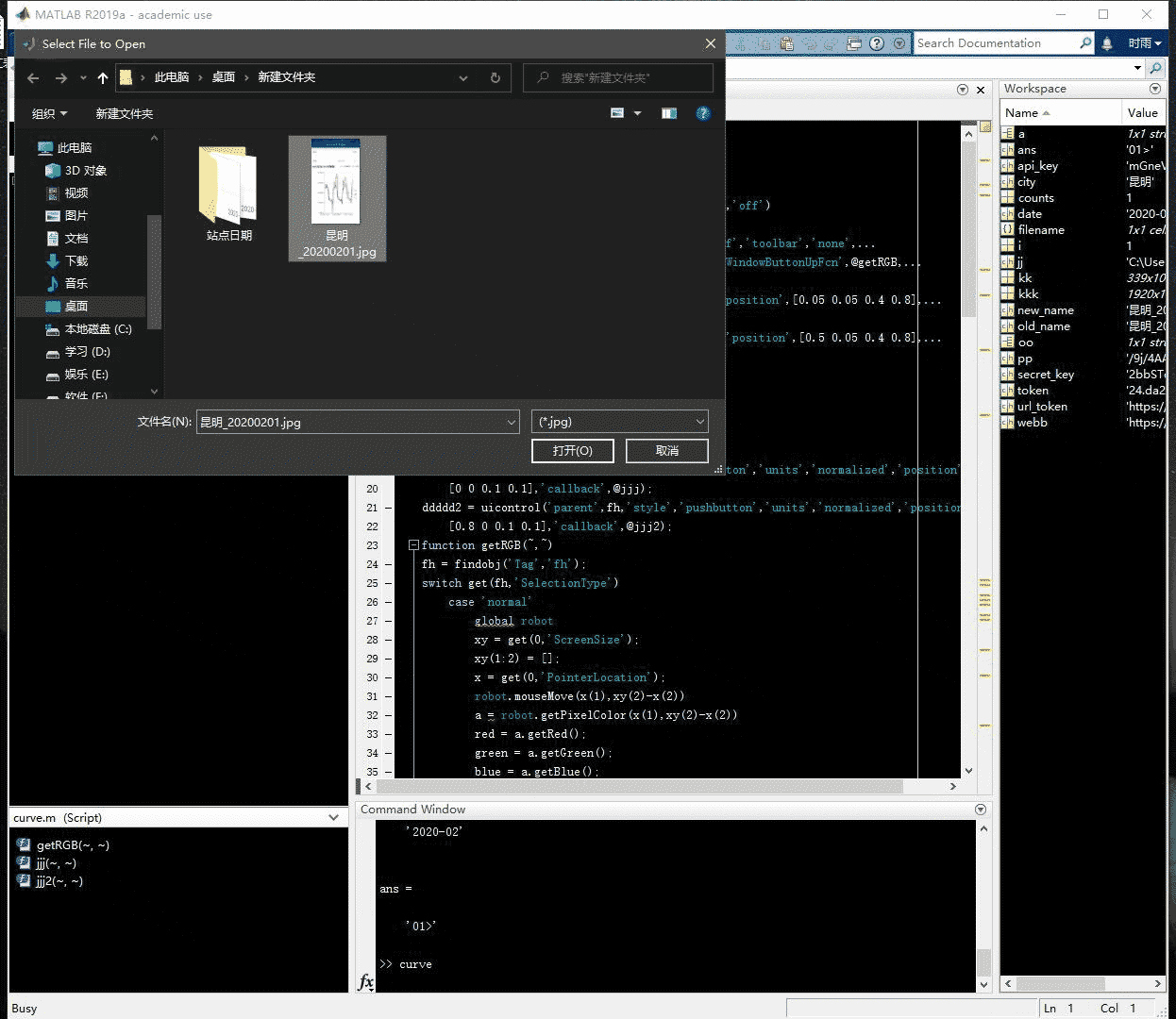解释一下，运行程序之后会提示你选中曲线图，然后会将图片显示出来，再单击需要提取的那条曲线，接着再右键，会对图片进行处理，去除多余的曲线。接下来再双击图片，会有十字出现用于选中坐标轴范围，先点左上角再点右下角就会出现提取到的曲线了，曲线的数据存储在代码中的变量里，可以自行处理，需要注意的是坐标轴的最大值和最小值需要自行在代码里面更改，因为我需要批量处理图片，所以都选取的固定值。

• 左下角的按钮是用来返回的，比如曲线没有提取好，可以点左下角按钮恢复。
• 右下角的按钮是用来继续的，在提取完一张图片之后，可以点右下角按钮继续选择图片

关于曲线提取的部分我稍微看了一下，效果还不错，代码也不难，思路建议去看原博主的，我这里主要提供这样一个工具方便批量处理图片曲线，并且支持多曲线提取。
代码如下：

import java.awt.Robot;
global robot
robot = java.awt.Robot;
close all
[ file path] = uigetfile('*.jpg','MultiSelect','off')
'color','w','position',[400 50 1400 800],'WindowButtonUpFcn',@getRGB,...
'Tag','fh');
Axes = axes('parent',fh,'units','normalized','position',[0.05 0.05 0.4 0.8],...
'visible','on')
Axes2 = axes('parent',fh,'units','normalized','position',[0.5 0.05 0.4 0.8],...
'visible','on')
axes(Axes);
imshow(a);
setappdata(fh,'im',a);
setappdata(fh,'Axes2',Axes2);
setappdata(fh,'Axes',Axes);
ddddd = uicontrol('parent',fh,'style','pushbutton','units','normalized','position',...
[0 0 0.1 0.1],'callback',@jjj);
ddddd2 = uicontrol('parent',fh,'style','pushbutton','units','normalized','position',...
[0.8 0 0.1 0.1],'callback',@jjj2);
function getRGB(~,~)
fh = findobj('Tag','fh');
switch get(fh,'SelectionType')
case 'normal'
global robot
xy = get(0,'ScreenSize');
xy(1:2) = [];
x = get(0,'PointerLocation');
robot.mouseMove(x(1),xy(2)-x(2))
a = robot.getPixelColor(x(1),xy(2)-x(2))
red = a.getRed();
green = a.getGreen();
blue = a.getBlue();
setappdata(fh,'RGB',[red green blue]);
case 'alt'
a = getappdata(fh,'im');
rgb = getappdata(fh,'RGB');
a1 = a(:,:,1);
a2 = a(:,:,2);
a3 = a(:,:,3);
d = find(abs(a1-rgb(1)>20)|abs(a2-rgb(2)>20)|abs(a3-rgb(3)>20));
a1(d) = 255;
a2(d) = 255;
a3(d) = 255;
a(:,:,1) = a1;
a(:,:,2) = a2;
a(:,:,3) = a3;
imshow(a)
setappdata(fh,'im2',a);
case 'open'
im = getappdata(fh,'im2');
im=rgb2gray(im);%灰度变化
thresh = graythresh(im);%二值化阈值
im=im2bw(im,thresh);%二值化
set(0,'defaultfigurecolor','w')
imshow(im)%显示图片
[y,x]=find(im==0);%找出图形中的“黑点”的坐标。该坐标是一维数据。
y=max(y)-y;%将屏幕坐标转换为右手系笛卡尔坐标
y=fliplr(y);%fliplr()——左右翻转数组
plot(x,y,'k.','Markersize', 2);
[Xx,Yy]=ginput(2);%Xx,Yy——指实际坐标框的两个顶点
min_x=0;
max_x=20;
min_y=0;
max_y=140;
x=(x-Xx(1))*(max_x-min_x)/(Xx(2)-Xx(1))+min_x;
y=(y-Yy(1))*(min_y-max_y)/(Yy(2)-Yy(1))+max_y;
plot(x,y,'k.','Markersize', 2);
axis([min_x,max_x,min_y,max_y])%根据输入设置坐标范围
rate_x=0.008;%曲线的最前端和最后段删除比例
rate_y=0.008;%曲线的最顶端和最底段删除比例
[x_uni,index_x_uni]=unique(x);%找出有多少个不同的x坐标
x_uni(1:floor(length(x_uni)*rate_x))=[];%除去前rate_x(如5%)的x坐标
x_uni(floor(length(x_uni)*(1-rate_x)):end)=[];%除去后rate_x的x坐标
index_x_uni(1:floor(length(index_x_uni)*rate_x))=[];%除去前rate_x的x坐标
index_x_uni(floor(length(index_x_uni)*(1-rate_x)):end)=[];%除去后rate_x的x坐标
[mxu,~]=size(x_uni);
[mx,~]=size(x);
for ii=1:mxu
if ii==mxu
ytemp=y(index_x_uni(ii):mx);
else
ytemp=y(index_x_uni(ii):index_x_uni(ii+1));
end
threshold1=mean(ytemp)-std(ytemp);
threshold2=mean(ytemp)+std(ytemp);
ytemp(find(ytemp<threshold1))=[];
ytemp(find(ytemp>threshold2))=[];
thresholdy=(max_y-min_y)*rate_y;
ytemp(find(ytemp>max_y-thresholdy))=[];
ytemp(find(ytemp<min_y+thresholdy))=[];
y_uni(ii)=mean(ytemp);
end
x_uni(find(isnan(y_uni)))=[];
y_uni(find(isnan(y_uni)))=[];
size(x_uni)
size(y_uni)
hh = find(x_uni<min_x);
x_uni(hh) = [];
y_uni(hh)= [];
Axes2 = getappdata(fh,'Axes2')
axes(Axes2)
plot(x_uni,y_uni)
title('提取曲线')
axis([min_x,max_x,min_y,max_y])%根据输入设置坐标范围
Axes = getappdata(fh,'Axes')
axes(Axes)
end
end
function jjj(~,~)
fh = findobj('Tag','fh');
a = getappdata(fh,'im');
imshow(a)
end
function jjj2(~,~)
[ file path] = uigetfile('*.jpg','MultiSelect','off')
fh = findobj('Tag','fh');
figure(fh)
imshow(a)
end

下面是相关的文章，有兴趣可以看看，有关MATLAB调用有关API的使用
链接: 百度云人脸识别.
链接: 百度云文字识别
链接: 有道翻译
链接: 百度地图经纬度.
链接: 百度云语音识别
链接: MATLAB 发送邮件 sendmail的使用.

展开全文• 在平滑光伏电站功率波动的应用场景下，提出一种储能系统典型功率曲线挖掘方法。基于储能系统功率在纵向时序的区间分布特性，利用基于Bloch球面的量子遗传算法寻找所有分布概率大于阈值的分布区间及相应特征功率值；...
• 描述了一种通用的在复杂特征图像中检测主曲线的新算法．基于曲线最短路径和方向偏移判据，主曲线检测的所有运算都在图形表达中进行．在图形网络搜寻中，采用了深度优先搜索方法．其应用背景是从地理测绘图中检测道路...
• 牙X线特征图像边缘曲线提取的MATLAB实现.pdf
• 基于特征曲线提取和自由曲面匹配的弹头自动比对建模.pdf
• 采用python，opencv开源库实现图片提取曲线数据 使用说明： openpic 可以选择采用自动采集测点数据或手动采集测点数据 ... 键盘o表示接受曲线提取结果，开始自动间隔采集计算曲线上点，并输出csv文件
• 步骤： 1.初始化： 假设有样本集为S，通过主成分分析，求取第一主成分直线，并窃取质心两边分别长度为3/2方差的长度，并计算每个点到直线的投影，以及距离。初始Voronoi区域也就只有一个，包括了所有点集。...

## 步骤：

### 1.初始化：

假设有样本集为S，通过主成分分析，求取第一主成分直线，并窃取质心两边分别长度为3/2方差的长度，并计算每个点到直线的投影，以及距离。初始Voronoi区域也就只有一个，包括了所有点集。

### 2.插入新线段：

1. 首先在点集中寻找离自己主成分线段最远的偏远点vf，同时改点周围还至少有2个点到该点距离小于到它们自己主成分线段的距离即：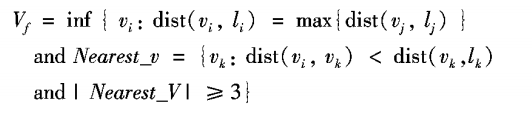其中i，j，k = 1, …,n, dist(vi， li)表示点到自己相应的主成分线段的距离。
假设本步骤开始样本区为S1，S2，… ，Sk，偏远点和他邻近点所形成的新样本区域为Sk+1。
2. Voronoi区域调整：
• 在S1，S2，… ，Sk，Sk+1找到有调整的样本区域，计算其第一主成分，并同上一步一样，计算得到新的主成分线段；
• 由于产生了新的主成分线段，对所有样本点调整它们的Voronoi区域。

### 3.构造和优化：

将上一步后得到的l1,l2,…,lk个线段构造Hamilton路径，该过程使用贪心算法：

• 令h=k,开始时有h个哈密顿子图，sub_HP。
• 当h>1，求每个子图的端点到其他子图端点的代价函数值，其定义: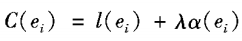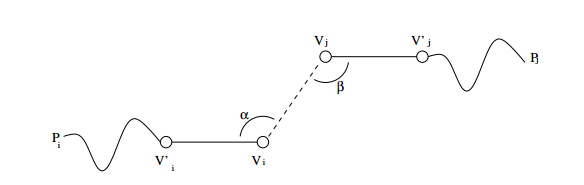e标示连接两个子图端点的边，l是边的长度，α是角度惩罚值，λ是角度惩罚系数；
• 连接使C值最小的边的端点，那么h=h-1，返回 上一步，直到h=1，该步即可产生所有子图的连线。
• 2-opt优化算法，对之前的Hamilton路径进行优化。

### 4.终止条件：

循环第2步和第3步，直到达到所设置的最大线段数，或者根据目标函数：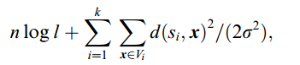计算的值达到最小即可停止。

## 测试结果：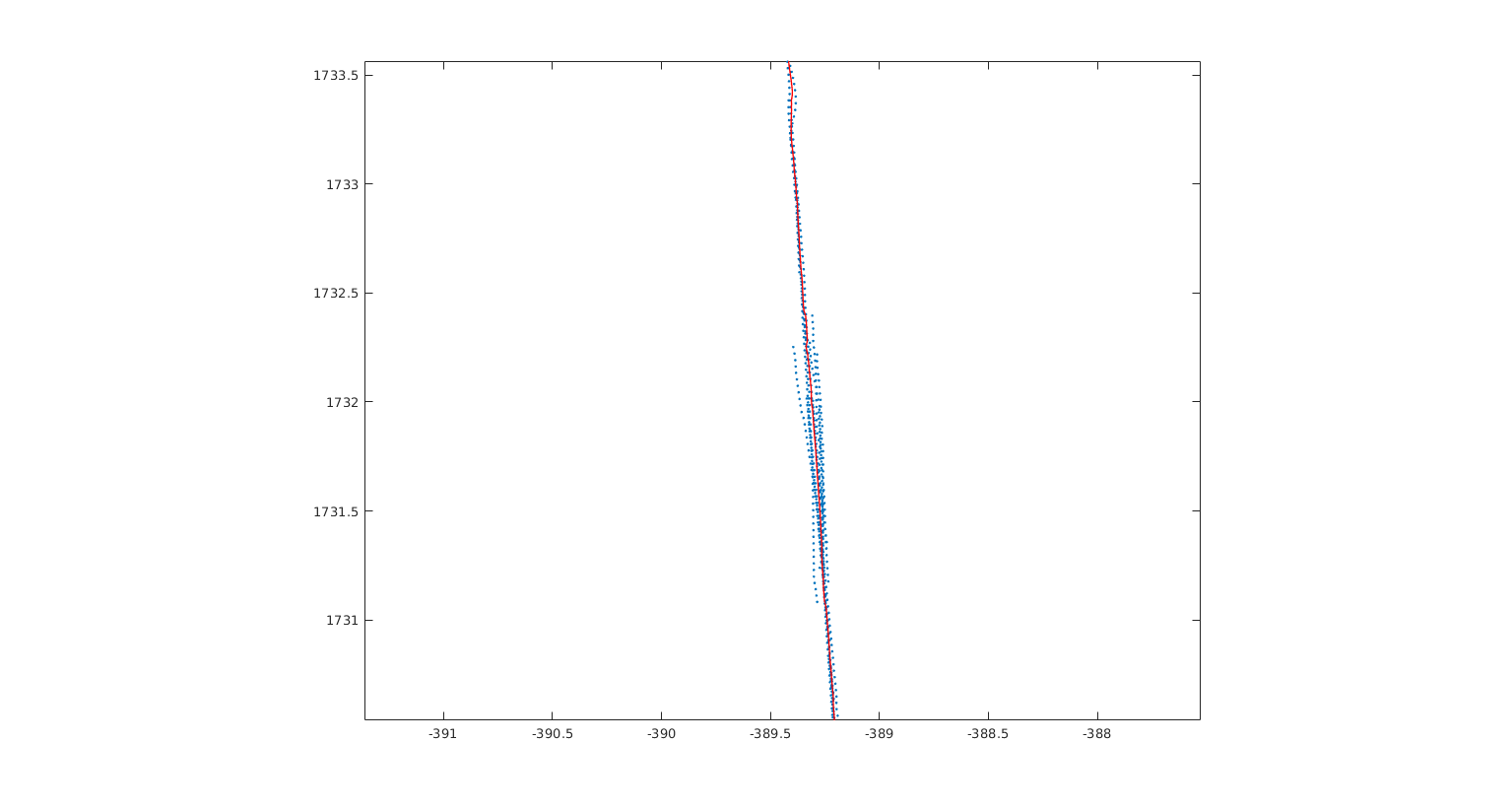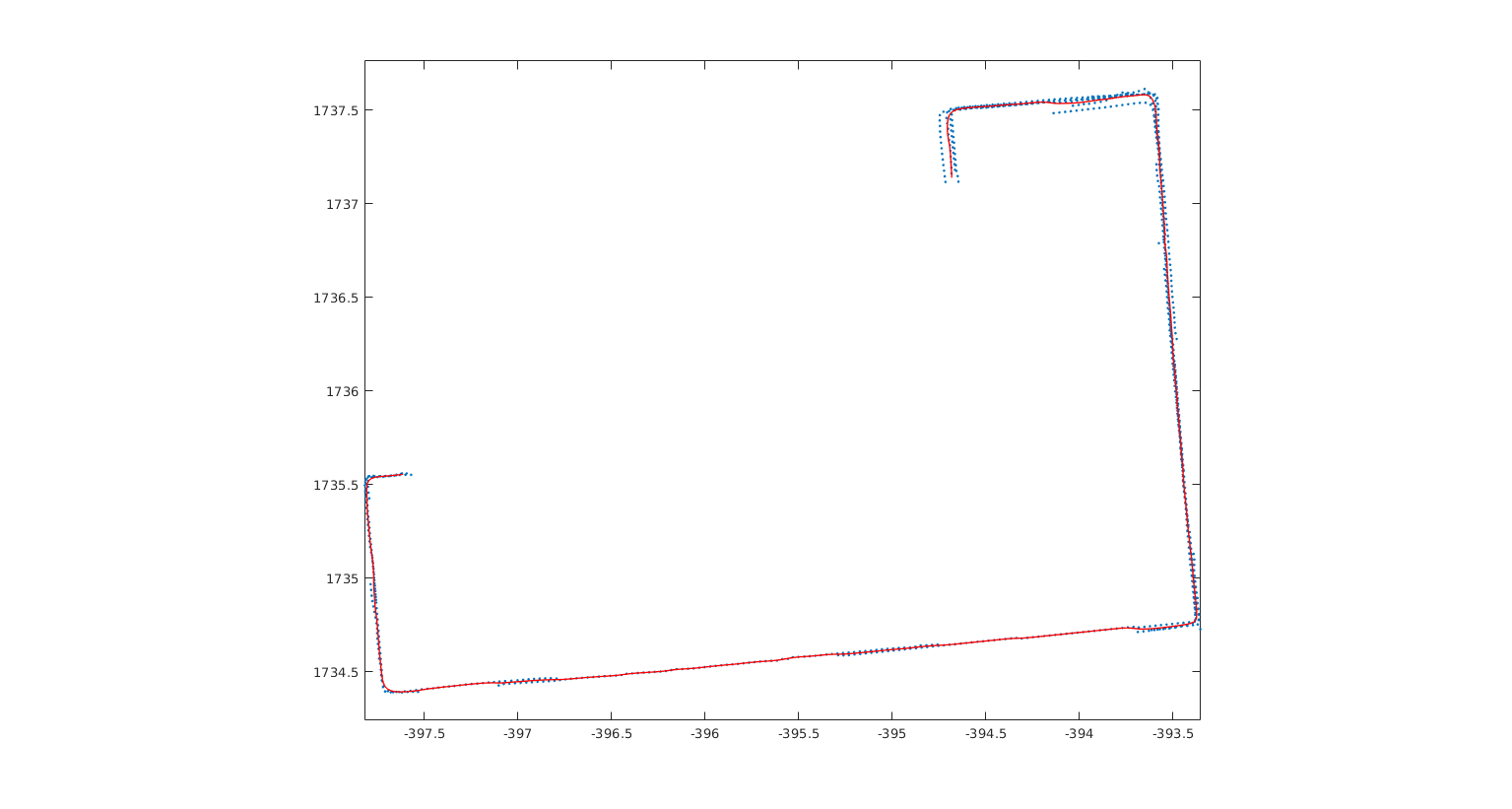展开全文算法
• 矢状面曲线形态是研究人体纵截面体型的前提和基础,也是提高服装合体性的关键。基于青年女性三维人体测量,利用逆向工程技术采集点云数据,并抽取表面轮廓曲线。用最小二乘法对采集到的数据进行拟合,得到青年女性正矢状...
• 然后用白色画笔覆盖，留一条曲线出来就行。第二步，打开getdata软件。第三步，打开图像。第四步，设置坐标。第五步，取出数据。第六步 导出数据 可以用getdata自带的，麻烦。提倡直接鼠标拉取想要的数据。拉取选好后...
• 行业资料-电子功用-基于业扩报装稳定周期的用电生长曲线提取系统和方法
• 网络游戏-基于SOM神经网络聚类算法的日负荷特征曲线提取方法.zip
•研究论文
• 研究小波变换提取瑞雷波频散曲线的具体方法 通过MATLAB编程实现小波变换提取瑞雷波频散曲线，同时应用于速度递增模型、含硬高速夹层和含软低速夹层等几个地层模型，对比理论频散曲线，验证该方法的可行性和实际工程...
• 运用基于图像特征的相对发射率数据处理...进一步对正常海水和油污染水体的光谱特性进行分析，通过反射率曲线比较，得出运用基于图像特征的反射率处理模型提取光谱信息和运用航空高光谱数据进行海上油污染检测的可行性。
• ## Maya曲线提取工具

千次阅读 2021-02-25 10:00:17
Maya曲线提取工具 零、前言 在Maya中通过脚本实现提取曲线的功能。 一、需求 美术在做Maya中做头发时如果每条头发都自己手动做会非常耗时。 希望可以选中某条曲线，然后进行复制，从而实现复用，提高工作效率。 ......